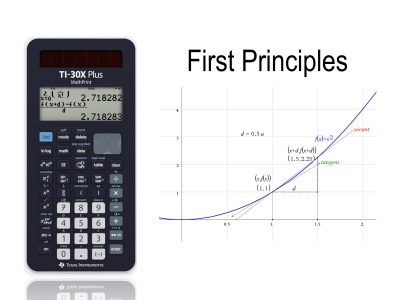# Activities

•• ##### AuthorAust Senior

45 Minutes

## First Principles#### Activity Overview

Students use the two function definition tools on the TI-30XPlus MathPrint calculator to neatly define the function f(x) and then g(x) for the gradient function using differentiation from first principles. By repeatedly decreasing the distance between the two points (limit) students can see the gradient also approaches a limit. This tool is then used to generate a list of gradient estimates with a view to establishing the rule for the gradient.

This activity includes a video tutorial.

#### Objectives

Students use function notation to express the gradient from first principles for a range of functions to explore the gradient at a point, followed by the general gradient of the function by generating a table of values.

#### Vocabulary

• First Principles